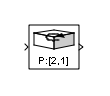Permute Dimensions

Rearrange dimensions of multidimensional array dimensions

• Library:

•Description

The Permute Dimensions block reorders the elements of the input signal by permuting its dimensions. You specify the permutation to be applied to the input signal using the Order parameter.

For example, to transpose a 3-by-5 input signal, specify the permutation vector [2 1] for the Order parameter. When you do, the block reorders the elements of the input signal and outputs a 5-by-3 matrix.

You can use an array of buses as an input signal to a Permute Dimensions block. For details about defining and using an array of buses, see Group Nonvirtual Buses in Arrays of Buses.

Ports

Input

expand all

This port accepts scalar, vector, matrix, and N-dimensional signals of any data type that Simulink® supports, including fixed-point, enumerated, and nonvirtual bus data types.

Data Types: half | single | double | int8 | int16 | int32 | int64 | uint8 | uint16 | uint32 | uint64 | Boolean | fixed point | enumerated | bus

Output

expand all

The block outputs the permutation of the input signal, according to the value of the Order parameter. The output has the same data type as the input.

Data Types: half | single | double | int8 | int16 | int32 | int64 | uint8 | uint16 | uint32 | uint64 | Boolean | fixed point | enumerated | bus

Parameters

expand all

Specify the permutation order to apply to the dimensions of the input signal. The value of this parameter must be an N-element vector where N is the number of dimensions of the input signal. The elements of the permutation vector must be a rearrangement of the values from 1 to N.

For example, the permutation vector [2 1] applied to a 5-by-3 input signal results in a 3-by-5 output signal, in other words, the transpose of the input signal.

Programmatic Use

 Block Parameter: Order Type: character vector Value: N-element vector Default: '[2 1]'

Block Characteristics

 Data Types Boolean | bus | double | enumerated | fixed point | half | integer | single Direct Feedthrough yes Multidimensional Signals no Variable-Size Signals yes Zero-Crossing Detection no